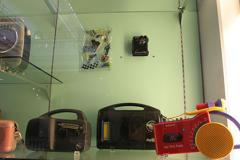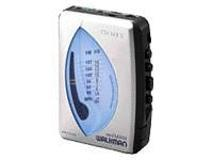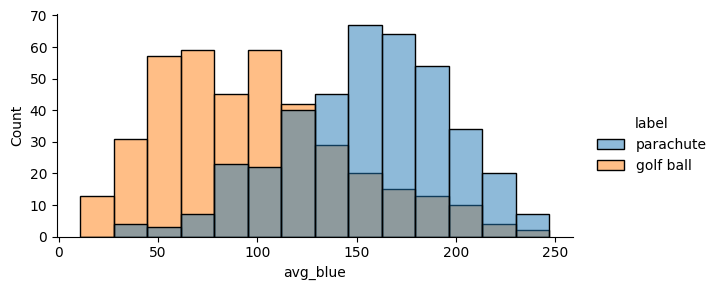# Tutorial 1: Data Frames (Wrangling an Image Dataset)¶

In this tutorial, we’ll learn how to use Meerkat to explore a dataset of images. We’ll use the `imagenette` dataset, a small 10-class subset of ImageNet.

Through this tutorial, you will learn about:

• the concept of data frames in Meerkat

• the different types of data that you can store in a Meerkat data frame

• how to use data frames to explore unstructured data

The content of this tutorial is provided in the `tutorial-0.ipynb` Jupyter notebook.

## 🔮 Importing Meerkat¶

Let’s start by importing Meerkat.

```import os
import meerkat as mk
import numpy as np
```

First, we’ll download some data to explore. We’re going to use the Imagenette dataset, a small subset of the original ImageNet. This dataset is made up of 10 classes (e.g. “garbage truck”, “gas pump”, “golf ball”).

In addition to downloading the data, `download_imagnette` prepares a CSV, `imagenette.csv`, with a row for each image.

```from meerkat.datasets.imagenette import download_imagenette

os.makedirs(dataset_dir, exist_ok=True)
```
```Extracting tar archive, this may take a few minutes...
```

Let’s take a look at the CSV.

```!head -n 5 downloads/imagenette2-160/imagenette.csv
```
```label,split,img_path
cassette player,train,train/n02979186/n02979186_9036.JPEG
cassette player,train,train/n02979186/n02979186_11957.JPEG
cassette player,train,train/n02979186/n02979186_9715.JPEG
cassette player,train,train/n02979186/n02979186_21736.JPEG
```

Next, we’ll load it into a Meerkat `DataFrame`.

## 📸 Creating an image `DataFrame`¶

For more information on creating DataFrames from various data sources, see the user guide section on I/O.

Meerkat’s core contribution is the DataFrame, a simple columnar data abstraction. The Meerkat DataFrame can house columns of arbitrary type – from integers and strings to complex, high-dimensional objects like videos, images, medical volumes and graphs.

We’re going to build a `DataFrame` out of the `imagenette.csv` file from the download above.

```# Create a `DataFrame`

# Create an `ImageColumn`` and add it to the `DataFrame`
df["img"] = mk.image(
df["img_path"],
base_dir=os.path.join(dataset_dir, "imagenette2-160")
)
df
```

The call to `head` shows the first few rows in the `DataFrame`. You can see that there are a few metadata columns, as well as the “img” column we added in.

## 🗂 Selecting data¶

When we create an `ImageColumn` we don’t load the images into memory. Instead, `ImageColumn` keeps track of all those filepaths we passed in and only loads the images when they are needed.

When we select a row of the `ImageColumn`, we get an instance `FileCell` back. A `FileCell` is an object that holds everything we need to materialize the cell (e.g. the filepath to the image and the loading function), but stops just short of doing so.

```img_cell = df["img"]
print(f"Indexing the `ImageColumn` returns an object of type: {type(img_cell)}.")
```
```Indexing the `ImageColumn` returns an object of type: <class 'meerkat.columns.deferred.file.FileCell'>.
```

To actually materialize the image, we simply call the cell.

```img = img_cell()
img
```We can subselect a batch of images by indexing with a slice. Notice that this returns a smaller `DataFrame`.

```imgs = df["img"][1:4]
print(f"Indexing a slice of the `ImageColumn` returns a: {type(imgs)}.")
imgs
```
```Indexing a slice of the `ImageColumn` returns a: <class 'meerkat.columns.deferred.file.FileColumn'>.
```
(FileColumn)
012The whole batch of images can be loaded together by calling the column.

```imgs();
```

One can load multiple rows using any one of following indexing schemes:

• Slice indexing: e.g. `column[4:10]`

• Integer array indexing: e.g. `column[[0, 4, 6, 11]]`

• Boolean array indexing: e.g. `column[np.array([True, False, False ..., True, False])]`

### 📎 Aside: `ImageColumn` under the hood, `DeferredColumn`.¶

If you check out the implementation of `ImageColumn` (at meerkat/column/image_column.py), you’ll notice that it’s a super simple subclass of `DeferredColumn`.

What’s a `DeferredColumn`? In `meerkat`, high-dimensional data types like images and videos are typically stored in a `DeferredColumn`. A `DeferredColumn` wraps around another column and lazily applies a function to it’s content as it is indexed. Consider the following example, where we create a simple `meerkat` column…

```  col = mk.column([0,1,2])
```

…and wrap it in a deferred column.

```  dcol = col.defer(fn=lambda x: x + 10)
dcol()  # the function is only called at this point!
```

Critically, the function inside a lambda column is only called at the time the column is called! This is very useful for columns with large data types that we don’t want to load all into memory at once. For example, we could create a `DeferredColumn` that lazily loads images…

```  >>> filepath_col = mk.PandasSeriesColumn(["path/to/image0.jpg", ...])
>>> img_col = filepath.defer(lambda x: load_image(x))
```

An `ImageColumn` is a just a `DeferredColumn` like this one, with a few more bells and whistles!

## 🛠 Applying operations over the DataFrame.¶

When analyzing data, we often perform operations on each example in our dataset (e.g. compute a model’s prediction on each example, tokenize each sentence, compute a model’s embedding for each example) and store them. The `DataFrame` makes it easy to perform these operations:

• Produce new columns (via `DataFrame.map`)

• Produce new columns and store the columns alongside the original data (via `DataFrame.update`)

• Extract an important subset of the datset (via `DataFrame.filter`).

Under the hood, dataloading is multiprocessed so that costly I/O doesn’t bottleneck our computation.

Let’s start by filtering the `DataFrame` down to the examples in the validation set.

```valid_df = df.filter(lambda split: split == "valid", is_batched_fn=True, batch_size=len(df))
```

### 🫐 Using `DataFrame.map` to compute average intensity of the blue color channel in the images.¶

To demonstrate the utility of the `map` operation, we’ll explore the relationship between the “blueness” of an image and the class of the image.

We’ll quantify the “blueness” of each image by simply computing the mean intensity of the blue color channel. This can be accomplished with a simple `map` operation over the `DataFrame`:

```blue_col = valid_df.map(
lambda img: np.array(img)[:, :, 2].mean(),
num_workers=2
)

# Add the intensities as a new column in the `DataFrame`
valid_df["avg_blue"] = blue_col
```

### 🪂 vs. ⛳️¶

Next, we’ll explore the relationship between blueness and the class label of the image. To do so, we’ll compare the blue intensity distribution of images labeled “parachute” to the distribution of of images labeled “golf ball”. Using the `seaborn` plotting package and our `DataFrame`, this can be accomplished in one line:

```## OPTIONAL: this cell requires the seaborn dependency: https://seaborn.pydata.org/installing.html
import seaborn as sns

plot_df = valid_df[np.isin(valid_df["label"], ["golf ball", "parachute"])]
sns.displot(
data=plot_df.to_pandas(),
x="avg_blue",
hue="label",
height=3,
aspect=2
)
```
```/home/runner/work/meerkat/meerkat/meerkat/dataframe.py:901: UserWarning: Could not convert column img of type <class 'meerkat.columns.deferred.file.FileColumn'>, it will be dropped from the output.
warnings.warn(
```
```<seaborn.axisgrid.FacetGrid at 0x7faaf89b0970>
``````valid_df["img"][int(np.argmax(valid_df["avg_blue"]))]()
```## 📉 ML with images in `meerkat`.¶

Let’s do some machine learning on our Imagenette `DataFrame`. We’ll take a resnet18 pretrained on the full ImageNet dataset, perform inference on the validation set, and analyze the model’s predictions and activations.

```import torch
from torchvision.models import resnet18
import torchvision.transforms as transforms
model = resnet18(weights="ResNet18_Weights.IMAGENET1K_V1")
```
```Downloading: "https://download.pytorch.org/models/resnet18-f37072fd.pth" to /home/runner/.cache/torch/hub/checkpoints/resnet18-f37072fd.pth
```
```  0%|          | 0.00/44.7M [00:00<?, ?B/s]
```
``` 37%|███▋      | 16.7M/44.7M [00:00<00:00, 174MB/s]
```
```100%|██████████| 44.7M/44.7M [00:00<00:00, 239MB/s]
```
```
```

### 💈 Applying a transform to the images.¶

In order to do inference, we’ll need to create a new `DeferredColumn`. The `ImageColumn` we defined above (i.e. `"img_path"`), does not apply any transforms after loading and simply returns a PIL image. Before passing the images through the model, we need to convert the PIL image to a `torch.Tensor` and normalize the color channels (along with a few other transformations).

Note: the transforms defined below are the same as the ones used by torchvision, see here.

In the cell below, we specify a transform when creating the `ImageColumn`.

```# Define transform
transform = transforms.Compose([
transforms.Resize(256),
transforms.CenterCrop(224),
transforms.ToTensor(),
transforms.Normalize(
mean=[0.485, 0.456, 0.406],
std=[0.229, 0.224, 0.225]),
])

# Create new column with transform
valid_df["input"] = valid_df["img"].defer(transform)
```

Notice that indexing this new column returns a `torch.Tensor`, not a PIL image…

```img = valid_df["input"]()
print(f"Indexing the `ImageColumn` returns an object of type: {type(img)}.")
```
```Indexing the `ImageColumn` returns an object of type: <class 'torch.Tensor'>.
```

… and that indexing a slice of this new column returns a `TensorColumn`.

```col = img = valid_df["input"][:3]()
print(f"Indexing a slice of the `ImageColumn` returns an object of type: {type(img)}.")
col
```
```Indexing a slice of the `ImageColumn` returns an object of type: <class 'meerkat.columns.tensor.torch.TorchTensorColumn'>.
```
(TorchTensorColumn)
0 torch.Tensor(shape=torch.Size([3, 224, 224]))
1 torch.Tensor(shape=torch.Size([3, 224, 224]))
2 torch.Tensor(shape=torch.Size([3, 224, 224]))

Let’s see what the full `DataFrame` looks like now.

```valid_df.head()
```
```---------------------------------------------------------------------------
TypeError                                 Traceback (most recent call last)
File /opt/hostedtoolcache/Python/3.9.17/x64/lib/python3.9/site-packages/IPython/core/formatters.py:922, in IPythonDisplayFormatter.__call__(self, obj)
920 method = get_real_method(obj, self.print_method)
921 if method is not None:
--> 922     method()
923     return True

File ~/work/meerkat/meerkat/meerkat/interactive/graph/marking.py:74, in unmarked.__call__.<locals>.decorate_context(*args, **kwargs)
71 @wraps(func)
72 def decorate_context(*args, **kwargs):
73     with self.clone():
---> 74         return reactive(func, nested_return=False)(*args, **kwargs)

File ~/work/meerkat/meerkat/meerkat/interactive/graph/reactivity.py:211, in reactive.<locals>.__reactive.<locals>.wrapper(*args, **kwargs)
209 # Call the function on the args and kwargs
210 with unmarked():
--> 211     result = fn(*unpacked_args, **unpacked_kwargs)
213 # TODO: Check if result is equal to one of the inputs.
214 # If it is, we need to copy it.
216 if _is_unmarked_context or _force_no_react or not any_inputs_marked:
217     # If we are in an unmarked context, then we don't need to create
218     # any nodes in the graph.
219     # `fn` should be run as normal.

File ~/work/meerkat/meerkat/meerkat/dataframe.py:193, in DataFrame._ipython_display_(self)
190     max_rows = meerkat.config.display.max_rows
191     df, formatters = self._repr_pandas_(max_rows=max_rows)
192     return display(
--> 193         HTML(df.to_html(formatters=formatters, max_rows=max_rows, escape=False))
194     )
196 return self.gui.table()._ipython_display_()

File /opt/hostedtoolcache/Python/3.9.17/x64/lib/python3.9/site-packages/pandas/core/frame.py:3129, in DataFrame.to_html(self, buf, columns, col_space, header, index, na_rep, formatters, float_format, sparsify, index_names, justify, max_rows, max_cols, show_dimensions, decimal, bold_rows, classes, escape, notebook, border, table_id, render_links, encoding)
3109 formatter = fmt.DataFrameFormatter(
3110     self,
3111     columns=columns,
(...)
3126     show_dimensions=show_dimensions,
3127 )
3128 # TODO: a generic formatter wld b in DataFrameFormatter
-> 3129 return fmt.DataFrameRenderer(formatter).to_html(
3130     buf=buf,
3131     classes=classes,
3132     notebook=notebook,
3133     border=border,
3134     encoding=encoding,
3135     table_id=table_id,
3137 )

File /opt/hostedtoolcache/Python/3.9.17/x64/lib/python3.9/site-packages/pandas/io/formats/format.py:1108, in DataFrameRenderer.to_html(self, buf, encoding, classes, notebook, border, table_id, render_links)
1099 Klass = NotebookFormatter if notebook else HTMLFormatter
1101 html_formatter = Klass(
1102     self.fmt,
1103     classes=classes,
(...)
1107 )
-> 1108 string = html_formatter.to_string()
1109 return save_to_buffer(string, buf=buf, encoding=encoding)

File /opt/hostedtoolcache/Python/3.9.17/x64/lib/python3.9/site-packages/pandas/io/formats/html.py:77, in HTMLFormatter.to_string(self)
76 def to_string(self) -> str:
---> 77     lines = self.render()
78     if any(isinstance(x, str) for x in lines):
79         lines = [str(x) for x in lines]

File /opt/hostedtoolcache/Python/3.9.17/x64/lib/python3.9/site-packages/pandas/io/formats/html.py:83, in HTMLFormatter.render(self)
82 def render(self) -> list[str]:
---> 83     self._write_table()
85     if self.should_show_dimensions:
86         by = chr(215)  # ×

File /opt/hostedtoolcache/Python/3.9.17/x64/lib/python3.9/site-packages/pandas/io/formats/html.py:258, in HTMLFormatter._write_table(self, indent)
--> 258 self._write_body(indent + self.indent_delta)
260 self.write("</table>", indent)

File /opt/hostedtoolcache/Python/3.9.17/x64/lib/python3.9/site-packages/pandas/io/formats/html.py:406, in HTMLFormatter._write_body(self, indent)
404 def _write_body(self, indent: int) -> None:
405     self.write("<tbody>", indent)
--> 406     fmt_values = self._get_formatted_values()
408     # write values
409     if self.fmt.index and isinstance(self.frame.index, MultiIndex):

File /opt/hostedtoolcache/Python/3.9.17/x64/lib/python3.9/site-packages/pandas/io/formats/html.py:401, in HTMLFormatter._get_formatted_values(self)
399 def _get_formatted_values(self) -> dict[int, list[str]]:
400     with option_context("display.max_colwidth", None):
--> 401         fmt_values = {i: self.fmt.format_col(i) for i in range(self.ncols)}
402     return fmt_values

File /opt/hostedtoolcache/Python/3.9.17/x64/lib/python3.9/site-packages/pandas/io/formats/html.py:401, in <dictcomp>(.0)
399 def _get_formatted_values(self) -> dict[int, list[str]]:
400     with option_context("display.max_colwidth", None):
--> 401         fmt_values = {i: self.fmt.format_col(i) for i in range(self.ncols)}
402     return fmt_values

File /opt/hostedtoolcache/Python/3.9.17/x64/lib/python3.9/site-packages/pandas/io/formats/format.py:895, in DataFrameFormatter.format_col(self, i)
893 frame = self.tr_frame
894 formatter = self._get_formatter(i)
--> 895 return format_array(
896     frame.iloc[:, i]._values,
897     formatter,
898     float_format=self.float_format,
899     na_rep=self.na_rep,
900     space=self.col_space.get(frame.columns[i]),
901     decimal=self.decimal,
903 )

File /opt/hostedtoolcache/Python/3.9.17/x64/lib/python3.9/site-packages/pandas/io/formats/format.py:1330, in format_array(values, formatter, float_format, na_rep, digits, space, justify, decimal, leading_space, quoting, fallback_formatter)
1314     digits = get_option("display.precision")
1316 fmt_obj = fmt_klass(
1317     values,
1318     digits=digits,
(...)
1327     fallback_formatter=fallback_formatter,
1328 )
-> 1330 return fmt_obj.get_result()

File /opt/hostedtoolcache/Python/3.9.17/x64/lib/python3.9/site-packages/pandas/io/formats/format.py:1363, in GenericArrayFormatter.get_result(self)
1362 def get_result(self) -> list[str]:
-> 1363     fmt_values = self._format_strings()
1364     return _make_fixed_width(fmt_values, self.justify)

File /opt/hostedtoolcache/Python/3.9.17/x64/lib/python3.9/site-packages/pandas/io/formats/format.py:1430, in GenericArrayFormatter._format_strings(self)
1428 for i, v in enumerate(vals):
1429     if not is_float_type[i] and leading_space or self.formatter is not None:
-> 1430         fmt_values.append(f" {_format(v)}")
1431     elif is_float_type[i]:
1432         fmt_values.append(float_format(v))

File /opt/hostedtoolcache/Python/3.9.17/x64/lib/python3.9/site-packages/pandas/io/formats/format.py:1410, in GenericArrayFormatter._format_strings.<locals>._format(x)
1407     return repr(x)
1408 else:
1409     # object dtype
-> 1410     return str(formatter(x))

File ~/work/meerkat/meerkat/meerkat/interactive/formatter/base.py:351, in DeferredFormatter.html(self, cell)
350 def html(self, cell: DeferredCell):
--> 351     return self.wrapped.html(cell())

File ~/work/meerkat/meerkat/meerkat/interactive/formatter/base.py:351, in DeferredFormatter.html(self, cell)
350 def html(self, cell: DeferredCell):
--> 351     return self.wrapped.html(cell())

TypeError: 'Tensor' object is not callable
```
```DataFrame(nrows: 5, ncols: 6)
```

### 💫 Computing model predictions and activations.¶

We’d like to perform inference and extract:

1. Output predictions

2. Output class probabilities

3. Model activations

Note: in order to extract model activations, we’ll need to use a PyTorch forward hook and register it on the final layer of the ResNet. Forward hooks are just functions that get executed on the forward pass of a `torch.nn.Module`.

```# Define forward hook in ActivationExtractor class
class ActivationExtractor:
"""Extracting activations a targetted intermediate layer"""

def __init__(self):
self.activation = None

def forward_hook(self, module, input, output):
self.activation = output

# Register forward hook
extractor = ActivationExtractor()
model.layer4.register_forward_hook(extractor.forward_hook);
```

We want to apply a forward pass to each image in the `DataFrame` and store the outputs as new columns: `DataFrame.map` is perfectly suited for this task.

```# 1. Move the model to GPU, if available
# device = 0
device = "cpu"
model.to(device).eval()

# 2. Define a function that runs a forward pass over a batch
def predict(input: mk.TensorColumn):
x: torch.Tensor = input.data.to(device)  # We get the underlying torch tensor with `data` and move to GPU
out: torch.Tensor = model(x)  # Run forward pass

# Return a dictionary with one key for each of the new columns. Each value in the
# dictionary should have the same length as the batch.
return {
"pred": out.cpu().numpy().argmax(axis=-1),
"probs": torch.softmax(out, axis=-1).cpu(),
"activation": extractor.activation.mean(dim=[-1,-2]).cpu()
}
# 3. Apply the update. Note that the `predict` function operates on batches, so we set
# `batched=True`. Also, the `predict` function only accesses the "input" column, by
# specifying that here we instruct update to only load that one column and skip others
pred_df = valid_df.map(function=predict, is_batched_fn=True, batch_size=32)
valid_df = mk.concat([valid_df, pred_df], axis="columns")
```

The predictions, output probabilities, and activations are now stored alongside the examples in the `DataFrame`.

```valid_df[["label_id", "input", "pred", "probs", "activation"]].head()
```
```---------------------------------------------------------------------------
KeyError                                  Traceback (most recent call last)
Cell In, line 1
----> 1 valid_df[["label_id", "input", "pred", "probs", "activation"]].head()

File ~/work/meerkat/meerkat/meerkat/interactive/graph/reactivity.py:211, in reactive.<locals>.__reactive.<locals>.wrapper(*args, **kwargs)
209 # Call the function on the args and kwargs
210 with unmarked():
--> 211     result = fn(*unpacked_args, **unpacked_kwargs)
213 # TODO: Check if result is equal to one of the inputs.
214 # If it is, we need to copy it.
216 if _is_unmarked_context or _force_no_react or not any_inputs_marked:
217     # If we are in an unmarked context, then we don't need to create
218     # any nodes in the graph.
219     # `fn` should be run as normal.

File ~/work/meerkat/meerkat/meerkat/dataframe.py:537, in DataFrame.__getitem__(self, posidx)
535 @reactive()
536 def __getitem__(self, posidx):
--> 537     return self._get(posidx, materialize=False)

File ~/work/meerkat/meerkat/meerkat/interactive/graph/marking.py:74, in unmarked.__call__.<locals>.decorate_context(*args, **kwargs)
71 @wraps(func)
72 def decorate_context(*args, **kwargs):
73     with self.clone():
---> 74         return reactive(func, nested_return=False)(*args, **kwargs)

File ~/work/meerkat/meerkat/meerkat/interactive/graph/reactivity.py:211, in reactive.<locals>.__reactive.<locals>.wrapper(*args, **kwargs)
209 # Call the function on the args and kwargs
210 with unmarked():
--> 211     result = fn(*unpacked_args, **unpacked_kwargs)
213 # TODO: Check if result is equal to one of the inputs.
214 # If it is, we need to copy it.
216 if _is_unmarked_context or _force_no_react or not any_inputs_marked:
217     # If we are in an unmarked context, then we don't need to create
218     # any nodes in the graph.
219     # `fn` should be run as normal.

File ~/work/meerkat/meerkat/meerkat/interactive/graph/reactivity.py:144, in reactive.<locals>.__reactive.<locals>.wrapper.<locals>._fn_outer_wrapper.<locals>._fn_wrapper(*args, **kwargs)
142 @wraps(_fn)
143 def _fn_wrapper(*args, **kwargs):
--> 144     return _fn(*args, **kwargs)

File ~/work/meerkat/meerkat/meerkat/dataframe.py:525, in DataFrame._get(self, posidx, materialize)
523 if not set(posidx).issubset(self.columns):
524     missing_cols = set(posidx) - set(self.columns)
--> 525     raise KeyError(f"DataFrame does not have columns {missing_cols}")
527 df = self._clone(data=self.data[posidx])
528 return df

KeyError: "DataFrame does not have columns {'label_id'}"
```

### 🎯 Computing metrics and analyzing performance.¶

Computing statistics on Meerkat `DataFrames` is straightforward because standard NumPy operators and functions can be applied directly to a `NumpyArrayColumn`. We take advantage of this below to compute the accuracy of the model.

```valid_df["correct"] = valid_df["pred"] == valid_df["label_idx"].data
accuracy = valid_df["correct"].mean()
print(f"Micro accuracy across the ten Imagenette classes: {accuracy:0.3}")
```

Furthermore, since the `DataFrame` is naturally converted to a Pandas DataFrame, it’s easy to use data visualization tools that interface with Pandas (e.g. seaborn, bokeh).

```## OPTIONAL: this cell requires the seaborn dependency: https://seaborn.pydata.org/installing.html
import seaborn as sns
import matplotlib.pyplot as plt
sns.barplot(data=valid_df.to_pandas(), y="label", x="correct");
```

### 🔎 Exploring model activations.¶

To better understand the behavior of our model, we’ll explore the activations of the final convolutional layer of the ResNet. Recall that when we performed our forward pass, we extracted these activations and stored them in a new column called `"activation"`.

Unlike the the `NumpyArrayColumn`s we’ve been working with so far, the activation column has an additional dimension of size 512.

To visualize the activations, we’ll use a dimensionality reduction technique (UMAP) to embed the activations in two dimensions. We’ll store these embeddings in two new columns “umap_0” and “umap_1”.

```## OPTIONAL: this cell requires the umap dependency: https://umap-learn.readthedocs.io/en/latest/
!pip install umap-learn
from umap import UMAP

# 1. Compute UMAP embedding
reducer = UMAP()
embs = reducer.fit_transform(valid_df["activation"])

# 2. Add the embedding to the DataFrame as two new columns
valid_df["umap_0"] = embs[:, 0]
valid_df["umap_1"] = embs[:, 1]

## OPTIONAL: this cell requires the seaborn dependency: https://seaborn.pydata.org/installing.html
import seaborn as sns
import matplotlib.pyplot as plt
sns.scatterplot(data=valid_df.to_pandas(), x="umap_0", y="umap_1", hue="label");
plt.legend(bbox_to_anchor=(1.05, 1), loc='upper left')
```

## 💾 Writing a `DataFrame` to disk.¶

Finally, we can write the updated `DataFrame`, with all the activations and predictions included, to disk for later use.

`DataFrame` size on disk: 25MB

On disk, the `DataFrame` is stored in a directory at the path passed to `DataFrame.write`. Within that directory, each column is stored separately. This allows us to read only a subset of columns from DataFrame on disk. Use the file explorer to the left to further expore the file structure of the `DataFrame`.

```valid_df
|  +-- meta.yml
|  +-- state.dill
|  +-- columns
|   +-- activation
|   +-- avg_blue
|   +-- correct
|   ...
|   +-- umap_1
```
```valid_df.write(os.path.join(dataset_dir, "valid_df"))
```
```valid_df = mk.read(os.path.join(dataset_dir, "valid_df"))
```